# Algebraic Identities

Go back to  'Algebra'

Explore the world of algebraic identities by going through its various aspects and properties. Find answers to questions like what are identities, how they are formed, easy ways to remember identities, commonly used algebraic identities, and discover more interesting facts around them.

Identity means that the left-hand side of the equation is identically equal to the right-hand side, for all values of the variables.

Let's understand algebraic identities in detail with this video.

 1 Algebraic Identities 2 Learn these along with Algebraic Identities 3 Solved Examples on Algebraic Identities 4 Interactive Questions  Questions on Algebraic Identities

 $$\mathbf{(x\!+\!a)\!(x\!+\!b) \!=\! x^2\!+\! (a\!+\!b)x\!+\!ab}$$ $$\mathbf{ (a+b)^2 = a^2 + 2ab + b^2}$$ $$\mathbf{ (a + b)(a - b) = a^2 - b^2}$$ $$\mathbf{ (a-b)^2 = a^2 - 2ab + b^2}$$

## Algebraic Identities

Algebraic identities are equations where the value of the left-hand side of the equation is identically equal to the value of the right-hand side of the equation.

Example:

Consider the equations:

$$5x-3 = 12$$  and  $$10x - 6 = 24$$

If you solve both equations separately, you will observe the value $$x = 3$$ in both cases.

If you write the equations in the form $$ax\: – b = c$$, you will see that the two equations are:

• $$ax\: – b = c$$
• $$2(ax \:– b) = 2c$$

Most equations in math work only for certain values.

Example: $$4x \:+ 5 = 17$$  is true only if  $$x\: = 3$$

Identities are useful because they are always true regardless of the value of the variables.

### Basic Algebraic Identities

It is very important that we learn about algebraic identities in maths.

The four most important algebraic identities or formulas are listed below.

 Algebraic Identities    \begin{align} &(a + b)^2 = a^2 + 2ab + b^2 \\ &(a - b)^2 = a^2 - 2ab + b^2 \\ &(a + b)(a − b) = a^2 − b^2 \\ &(x\!+\! a) (x\!+\! b) = x^2 \!+\! x(a\!+\!b) \!+\! ab \end{align}

Apart from these simple algebraic identities listed above, there are other algebraic identities which we will use in higher grades.

Check them on the page Algebraic Identities Formula and Examples.

## Learn These Along with Algebraic Identities!

Here are a few more lessons related to algebraic identities.

These topics will not only help you master the concept for algebraic identities, but also other topics related to it.

 Click on any of the short lessons you want to explore! Variable Expressions Algebraic Expressions Algebraic Formulas Linear Equations Exponents Polynomials Quadratic Equations

### Proof of $$\mathbf{(x\!+\!a)\!(x\!+\!b) \!=\! x^2\!+\! (a\!+\!b)x\!+\!ab}$$

$$(x+a)(x+b)$$ is nothing but the area of a rectangle whose sides are $$x+a$$ and $$x+b$$.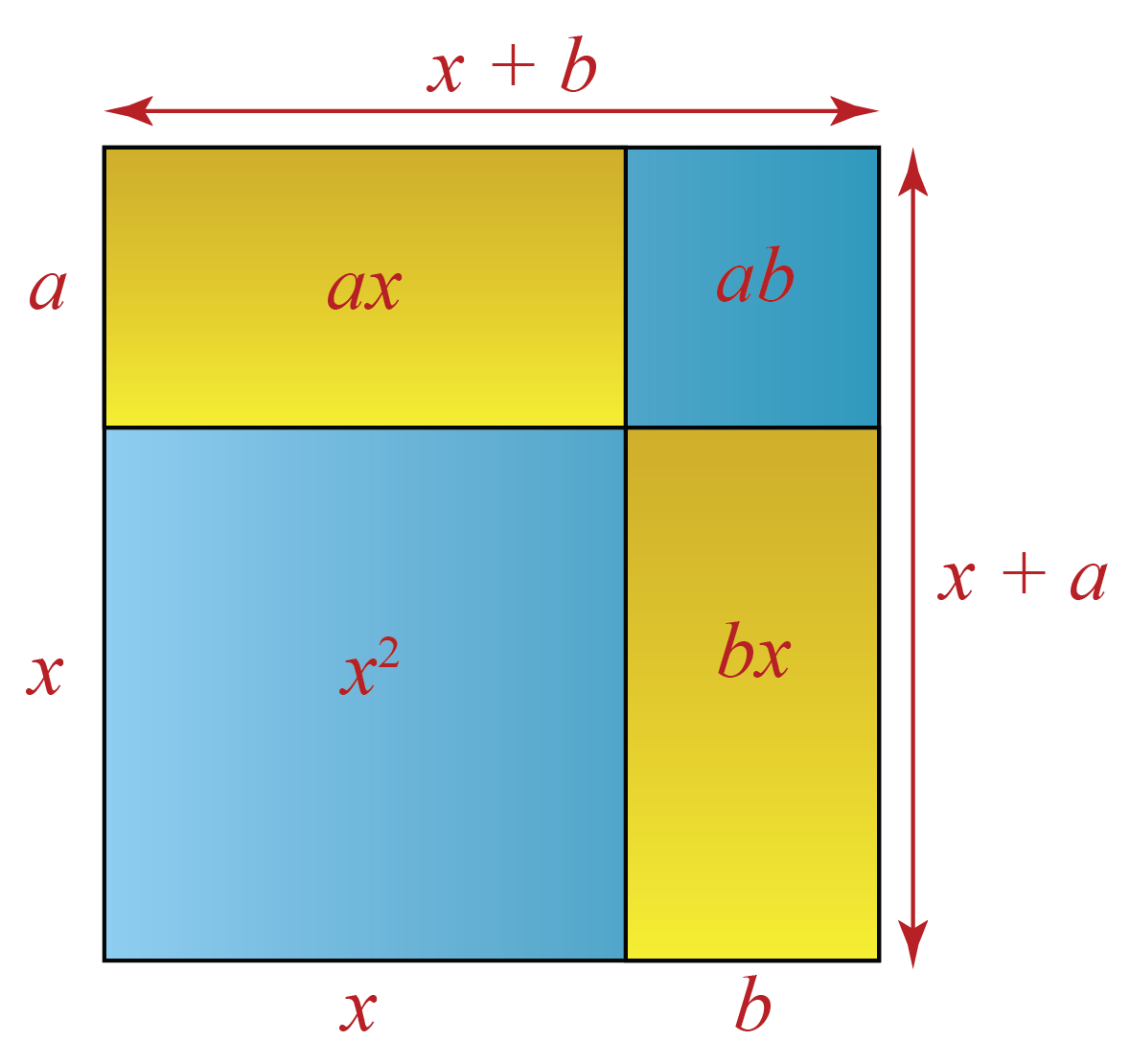The area of a rectangle with sides $$x+a$$ and $$x+b$$ in terms of the individual areas of the rectangles and the square is:

\begin{align} &x^2 +ax+bx+b^2 \\ &x^2 + (a+b)x+b^2 \end{align}

 $$\therefore (x+a)(x+b) = x^{2}+ (a+b)x+a b$$

You can use the following simulation to understand this visually.

### Proof of $$\mathbf{ (a+b)^2 = a^2 + 2ab + b^2}$$

$$(a+b)^2$$ is nothing but $$(a+b) \times (a+b)$$.

This can be visualized as a square whose sides are $$(a+b)$$and area is $$(a+b)^2$$.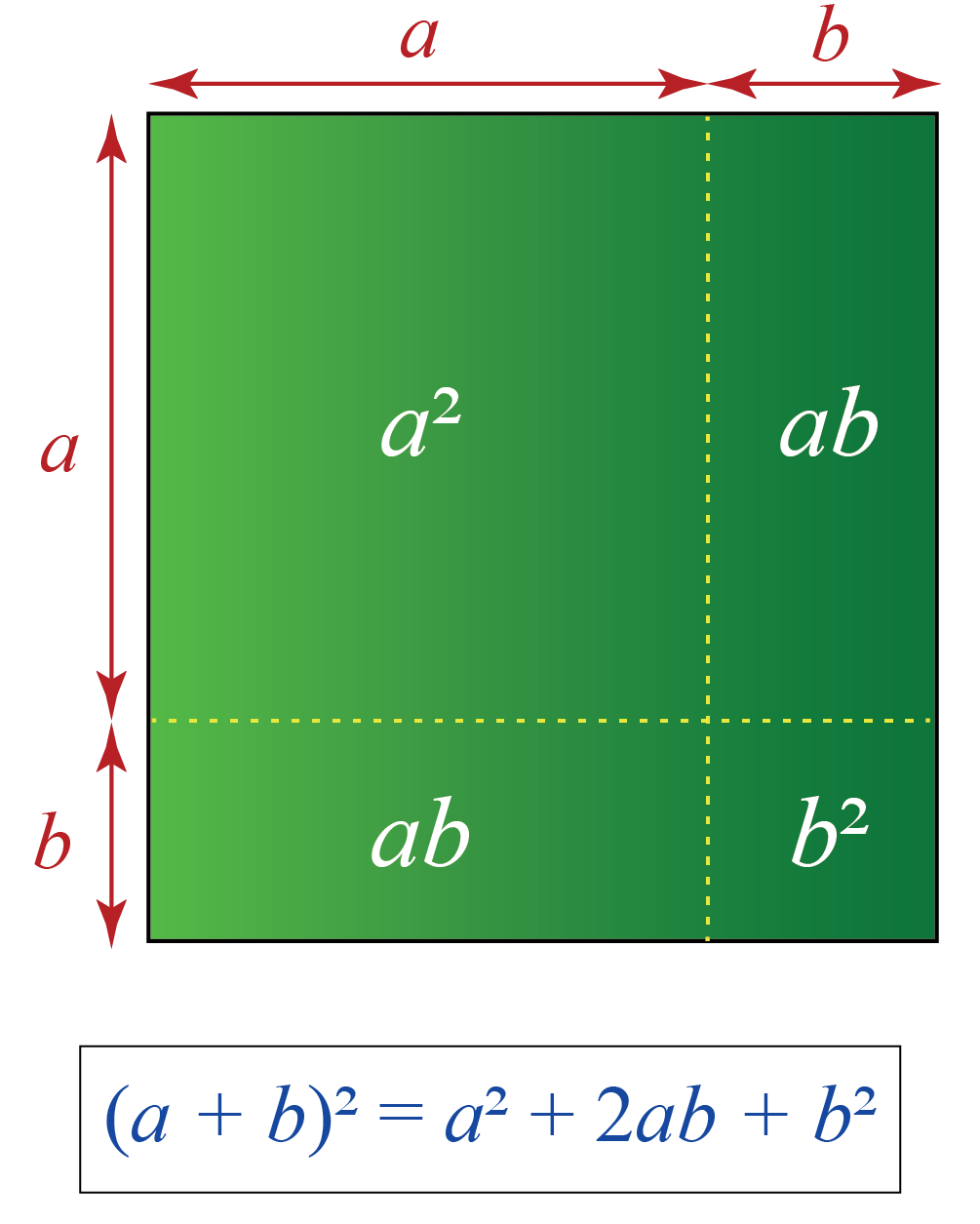Area of the square $$(a+b)^2$$ in terms of the product is $$(a+b)(a+b)$$.

The area of the square $$(a+b)^2$$ is also equal to the sum of the areas of the individual squares and rectangles.

Therefore,

 $$\therefore (a+b)^2 = (a)^2+(b)^2 +2ab$$

Do you find this hard to understand?

You can use the following simulation to understand this visually.

### Proof of $$\mathbf{ (a + b)(a - b) = a^2 - b^2}$$

(a + b) (a - b) can be visualised as the area of a rectangle whose sides are (a + b) and (a - b).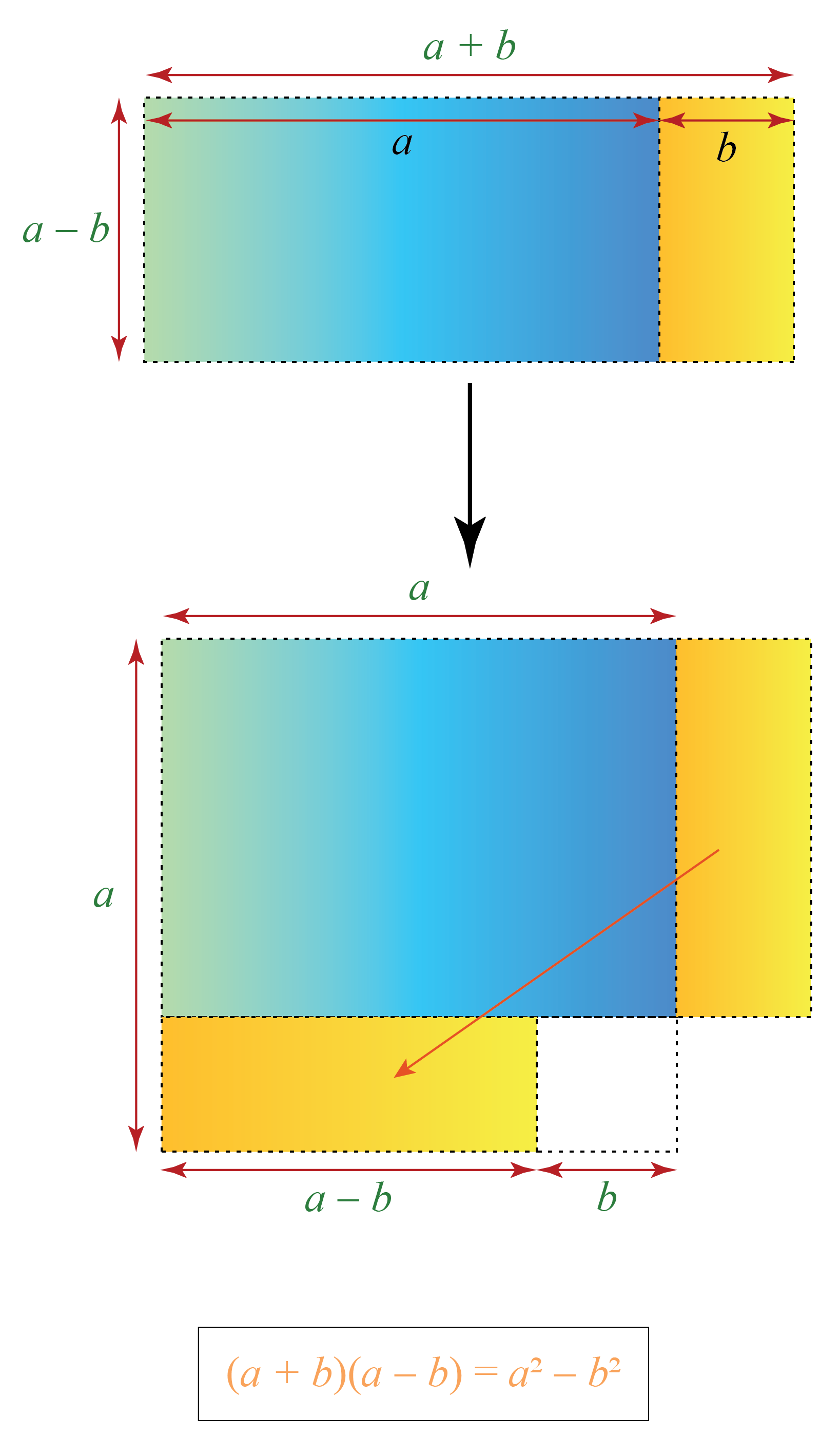Re-arranging the individual squares and rectangles, we get:

 $$(a+b)(a-b) =a^{2}-b^{2}$$

Are you confused?

### Proof of $$\mathbf{ (a-b)^2 = a^2 - 2ab + b^2}$$

Once again, let’s think of \begin{align} (a - b)^2 \end{align} as the area of a square with length \begin{align} (a - b) \end{align}.

To understand this, let's begin with a large square of area $$a^2$$.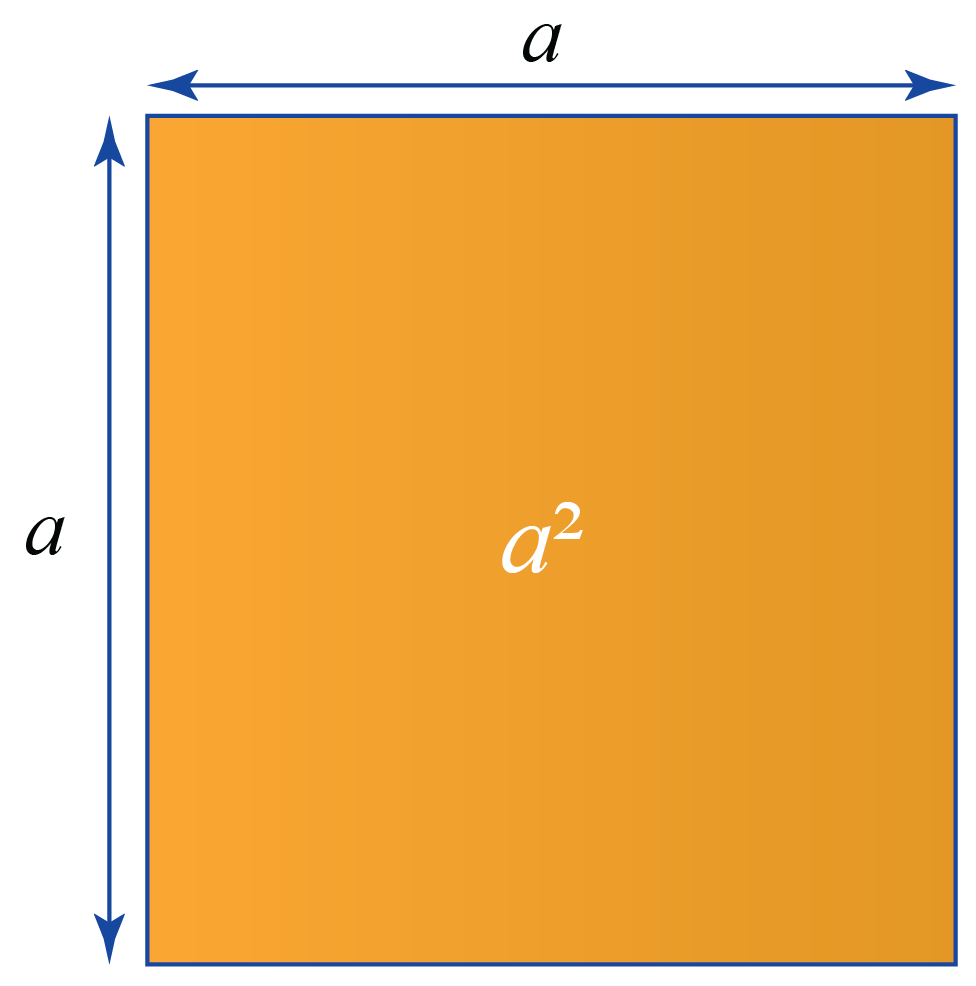We will reduce the length of all sides by $$b$$.

We now have to remove the extra bits from $$a^2$$ to be left with \begin{align} (a - b)^2 \end{align}.

In the figure below, \begin{align} (a - b)^2 \end{align} is shown by the blue area.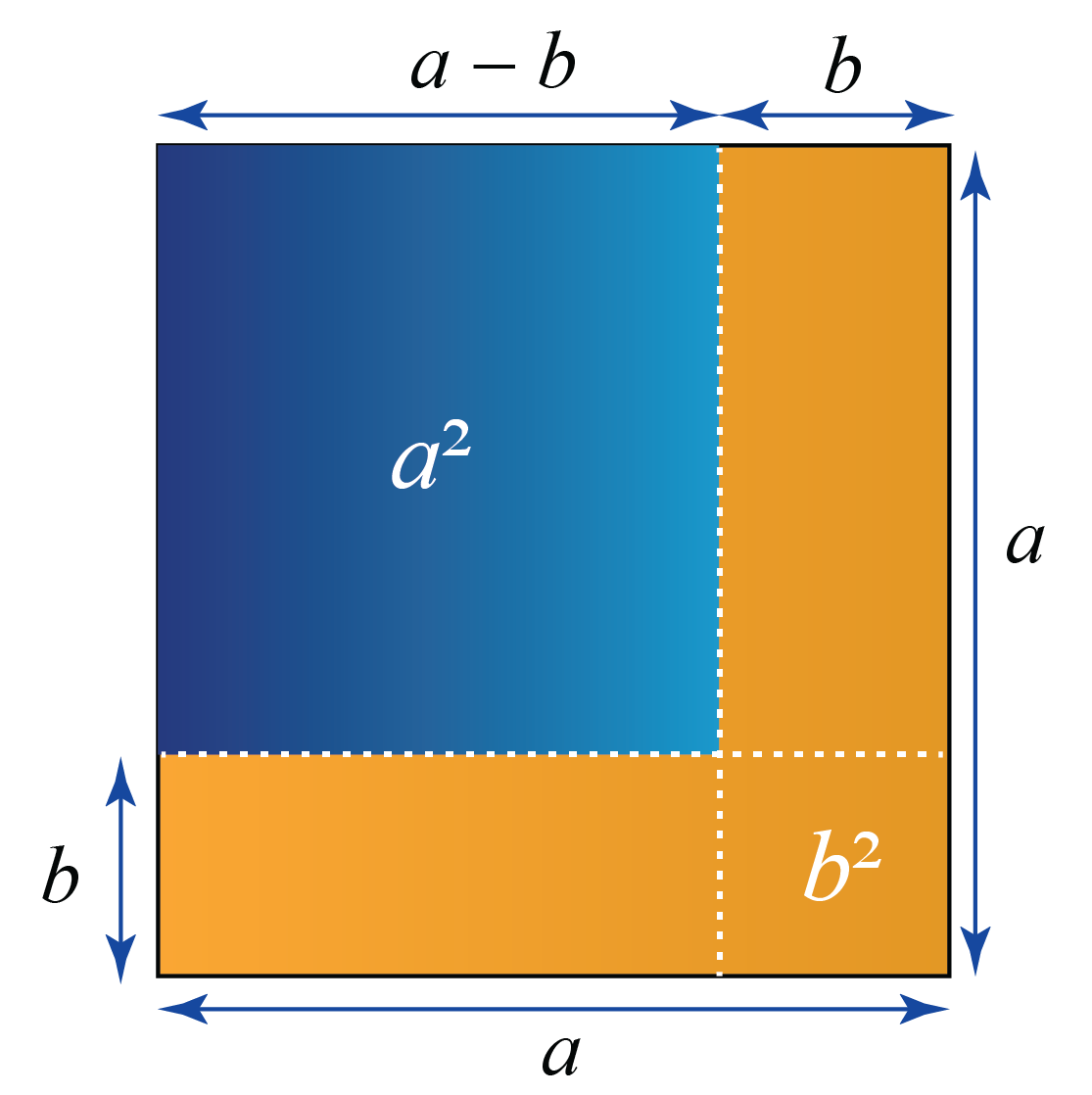To get the blue square from the larger orange square, we have to subtract the vertical and horizontal strips that have the area $$ab$$.

However, removing $$ab$$ twice will also remove the overlapping square at the bottom right corner twice.

Hence, we add $$b^2$$.

\begin{align} (a − b)^2 = a^2 − ab − ab + b^2 \end{align}

 $$\therefore (a-b)^2= a^2 -2ab+b^2$$

### Algebraic Identities - Calculator

The calculator below will help you solve sums based on the identities $$(a-b)^2, \; (a + b) \; (a - b), \; (a+b)^2$$

Enter the values of $$a$$ and $$b$$ in the simulation below.

## Algebraic Identities Problems - Solved Examples

Let's look at a few examples of algebraic identities now.

 Example 1

Using algebraic identities, find $$(2x + 3y)^2$$.

Solution:

This is similar to the LHS of the identity:

\begin{align} (a + b)^2 = a^2 + 2ab + b^2 \end{align}

where $$( a$$ is $$2x$$ and $$b$$ is $$3y)$$

\begin{align} (2x + 3y)^2 &= (2x)^2 + 2(2x)(3y) + (3y)^2 \\ &= 4x^2 + 12xy + 9y^2 \end{align}

 $$(2x \!+\! 3y)^2\!=\! 4x^2\! +\! 12xy \!+\! 9y^2$$
 Example 2

Using identities, solve $$297 \times 303$$

Solution:

$$297 \times 303$$ can be written as $$( 300 - 3 ) \times ( 300 + 3 )$$

This is based on the identity:

\begin{align} (a + b)(a − b) = a^2 − b^2 \end{align}

where

$$a = 300$$ and $$b = 3$$

Substituting the values in the above identity, we get:

\begin{align} (300 - 3)(300 + 3) &= 300^2 − 3^2 \\ &= 90000 - 9 \\ &= 89991 \end{align}

 $$\therefore \;297 \times 303 =89991$$
 Example 3

Expand $$( pqr + 2 )( pqr - 5 )$$

Solution:

This is based on the identity:

\begin{align} (x+ a) (x+ b) = x^2 + x(a+b) + ab \end{align}

where

$$x = pqr$$ and $$a = 2$$ and $$b = -5$$

Substituting in the above equation:

\begin{align} &( pqr + 2 )( pqr - 5 ) \\&= (pqr)^2 \!+\! pqr(2 + (-5)) \!+ \!(2)(-5) \\ &= p^2q^2r^2 -3pqr -10 \end{align}

 $$(pqr \!+\! 2 )( pqr \!- \!5 )\!= \! p^2q^2r^2 \!-\!3pqr\! -\!10$$
 Example 4

Simplify $$( 7x +4y )^2 + ( 7x - 4y )^2$$

Solution:

To solve this, we need to use the following identities:

\begin{align} (a + b)^2 = a^2 + 2ab + b^2 \end{align}

\begin{align} (a - b)^2 = a^2 - 2ab + b^2 \end{align}

Here,

$$a = 7x$$ and $$b = 4y$$

Substituting the values, we get:

\begin{align} &( 7x +4y )^2 + ( 7x - 4y )^2 \\ &= ( 49x^2 + 56xy + 16y^2)\\ &+ (49x^2 - 56xy + 16y^2) \\ &= 98x^2 + 32y^2 \end{align}

 $$(7x \!+\!4y )^2 \!\!+\! (7x\! -\! 4y)^2 \!\!=\!98x^2 \!+\! 32y^2$$
 Example 5

The area of a square is $$9x^2 +12x +4$$

What is the measure of its side?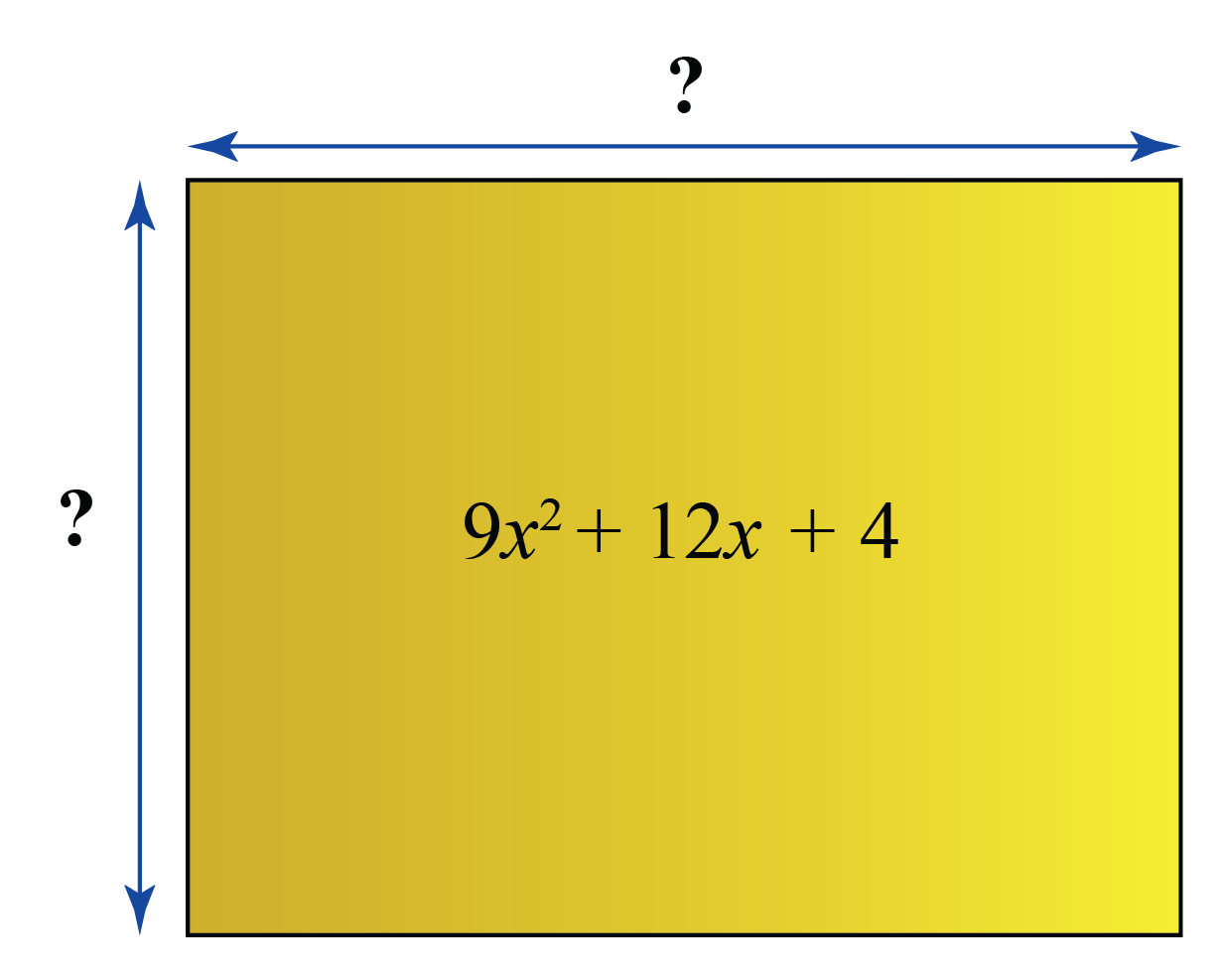Solution:

This looks like the RHS of the identity:

\begin{align} (a+b)^2 = a^2 +2ab+b^2 \end{align}

Where,

\begin{align} a^2 &= 9x^2 \\ \Rightarrow a &= 3x \\ b^2&=4\\ \Rightarrow b&=2 \\ 2 \times 3x \times 2 &= 2ab \end{align}

Therefore, Area of the square in terms of the product of its sides is the LHS of the identity $$(a+b)^2$$

 $$\therefore$$ Side of the square $$= (3x+2)$$

## Interactive Questions on Algebraic Identities

Here are a few problems on algebraic identities for you to practice.Challenging Questions
1. If $$x + \frac{1}{x} = 6$$, find the value of $$x^2 + \frac{1}{x^2}$$
2. Find the value of $$a-b$$, if $$(a+b) = 5$$ and $$ab = 4$$
3.  The length and breadth of a rectangle measures $$2x + 3$$ units and $$2x - 3$$ units. Find the area of the rectangle in terms of $$x$$

## Summary

The magic of math lies in the amazing concepts that it is built upon. At Cuemath, we explore and interact with these concepts in a fun way!

The math journey around algebraic identities numbers starts with what a student already knows, and goes on to creatively crafting a fresh concept in the young minds. Done in a way that not only it is relatable and easy to grasp, but also will stay with them forever.

The best part, this isn’t the end. With a universe built around algebraic identities numbers at Cuemath, one can take their math journey forward with solved examples, practice questions, quizzes, worksheets, practice papers, and so much more. Again, all of it, exclusively around algebraic identities.

## 1. What are the four algebraic identities?

The four commonly used algebraic identities are given below:

• Square of the sum of two binomials
\begin{align} (a+b)(a+b) = (a + b)^2 = a^2 + 2ab + b^2 \end{align}
• Square of the difference of two binomials
\begin{align}(a-b)(a-b) = (a - b)^2 = a^2 - 2ab + b^2 \end{align}
• Product of the sum and the difference of two binomials
\begin{align} (a + b)(a − b) = a^2 − b^2 \end{align}
• Product of two binomials
\begin{align} (𝑥 + a) (x+ b) = x^2 + x(a+b) + ab \end{align}

Use the Algebraic Identities Calculator to solve some algebraic identities problems .

## 2. How can I learn algebraic identities?

• Algebraic identities can be easily memorized by visualising the identities as squares or rectangles.
• They can also be remembered by the factored forms rather than the simplified forms.

## 3. What is the formula of identity?

An identity is an equation that is always true regardless of the value that is assigned to the variable.

The simple algebraic identities or formulas are:

\begin{align} &(a + b)^2 = a^2 + 2ab + b^2 \\ &(a - b)^2 = a^2 - 2ab + b^2 \\ &(a + b)(a − b) = a^2 − b^2 \\ &(x\!+\! a) (x\!+\! b) = x^2 \!+\! x(a\!+\!b) \!+\! ab \end{align}

More Important Topics
Numbers
Algebra
Geometry
Measurement
Money
Data
Trigonometry
Calculus